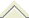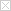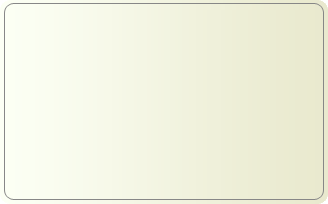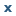• 小小坏人的：
• 博客首页
• 博文--

aa:=EMA(C,5);bb:=EMA(C,10);cc:=EMA(C,20);dd:=EMA(C,30);ee:=EMA(C,60);M1:=1000*bb/cc＜=1115 AND 1000*bb/cc＞=875;M2:=1000*cc/dd＜=1120 AND 1000*...